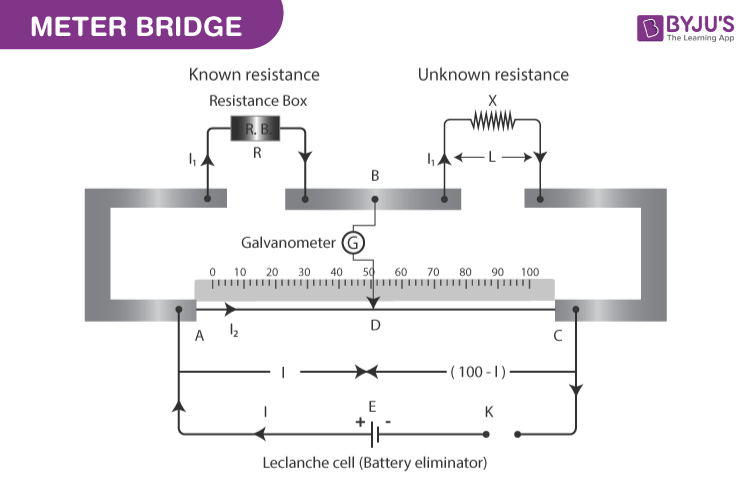# A resistance R is to be measured using a meter bridge. Student chooses the standard resistance S to be 100Ω. He finds the null point at l1 = 2.9cm. He is told to attempt to improve the accuracy. Which of the following is a useful way? (1) He should measure l1 more accurately (2) He should change S to 1000Ω and repeat the experiment (3) He should change S to 3Ω and repeat the experiment (4) He should give up hope of a more accurate measurement with a meter bridge

Answer: (3) He should change S to 3Ω and repeat the experiment

The balance of a metre bridge is always maintained. The long wire in a metre bridge measures 1m = 100cm in length and is composed of constantan or manganin with a uniform cross-sectional area. The formulae that were employed in the solution are given here.

$$\begin{array}{l}frac{l_{1}}{100 – l_{1}} = frac{R}{S}\end{array}$$

## Meter bridge

A meter bridge also called a slide wire bridge is an instrument that works on the principle of a Wheatstone bridge. A meter bridge is used in finding the unknown resistance of a conductor as that of in a Wheatstone bridge. The devices required in finding the unknown resistance of a conductor using a meter bridge are:

• Meter bridge
• Resistance box
• Galvanometer
• Unknown Resistance of a length 1 m
• Screw gauge
• Connecting Wires
• Jockey
• One way key

### How is a meter bridge used in finding the unknown resistance?

A meter bridge is an apparatus utilized in finding the unknown resistance of a coil. The below figure is the diagram of a useful meter bridge instrument.In the above figure,

• R = Resistance
• P = Resistance coming across AB
• S = Unknown Resistance
• Q = Resistance between the joints BD

AC is the long wire measuring 1m in length and it is made of constantan or manganin having a uniform area of the cross-section such that

L1 + L2 = 100

Assuming that

• L1 = L
• L2 = 100 – L

Relation obtains the unknown resistance ‘X’ of the given wire:

X = RL2/L1

X = R(100 – L)/L

### Given parameters

• S = 100Ω
• l1 = 2.9 cm

$$\begin{array}{l}frac{2.9}{100 – 2.9} = frac{R}{100}\end{array}$$
.

$$\begin{array}{l}frac{2.9}{97.1} = frac{R}{100}\end{array}$$
.

97.1R = 290

R = 290/97.1

R = 2.98Ω

2.98Ω ≈ 3Ω

The meter-bridge is of the same order, as a result, the student must use 3Ω to improve the accuracy of the measurement of resistance R.(0)(0)